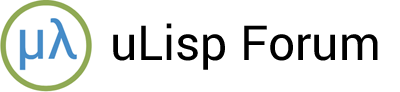# Extracting bitfields

#1

Here’s a useful routine bits I wrote for the uLisp RISC-V assembler, but it has applications in programming for microcontroller boards such as the Arduino. It extracts a single bit, or a bit field of several bits, from the number provided as its first parameter:

``````(defun bits (x a &optional b)
(if b (logand (ash x (- b)) (1- (ash 1 (- a b -1))))
(logand (ash x (- a)) 1)))
``````

To extract a single bit provide the bit position of the bit as the second argument; for example:

``````> (bits #b1001011001010011 4)
1
``````

To extract a bitfield, specify the starting and ending bit positions, inclusive; for example:

``````> (format t "~b" (bits #b1001011001010011 6 2))
10100
``````

Note that I’m taking advantage of the ~b format directive added in uLisp 3.5 to show the result in binary.

#2

Looks similar to `ldb`, although that doesn’t have the reversing ability.

`ldb` and `dpb` are CL functions that would definitely be useful in uLisp.

#3

I’ll need to read about `ldb` and `dpb` - thanks!

Just realised bits can be written more simply:

``````(defun bits (x a &optional (b a))
(logand (ash x (- b)) (1- (ash 1 (- a b -1)))))
``````

#4

`ldb` and `dpb`, for reference.

They sorta-depend on the additional function `byte`, because they take an implementation-defined representation of what a byte is. (CL had a lot of legacy stuff to contend with.) SBCL uses a simple cons cell, though, which I imagine is inherited from CMUCL.

#5

It looks like:

``````(ldb (byte length position) arg)
``````

is equivalent to my:

``(bits arg (+ position length -1) position)``